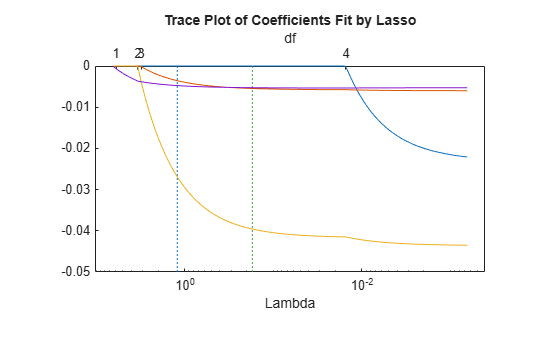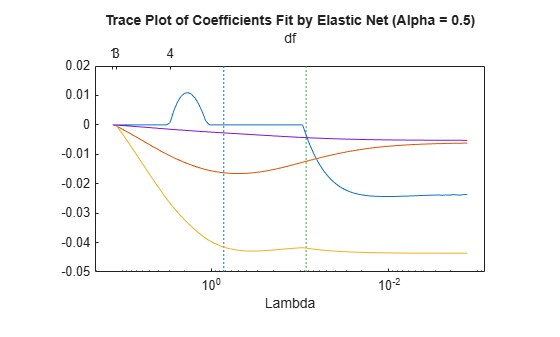Documentation

### This is machine translation

Mouseover text to see original. Click the button below to return to the English version of the page.

## Lasso and Elastic Net with Cross Validation

This example shows how to predict the mileage (MPG) of a car based on its weight, displacement, horsepower, and acceleration, using the lasso and elastic net methods.

Load the `carbig` data set.

`load carbig`

Extract the continuous (noncategorical) predictors (lasso does not handle categorical predictors).

`X = [Acceleration Displacement Horsepower Weight];`

Perform a lasso fit with 10-fold cross validation.

`[b fitinfo] = lasso(X,MPG,'CV',10);`

Plot the result.

`lassoPlot(b,fitinfo,'PlotType','Lambda','XScale','log');`Calculate the correlation of the predictors. Eliminate NaNs first.

```nonan = ~any(isnan([X MPG]),2); Xnonan = X(nonan,:); MPGnonan = MPG(nonan,:); corr(Xnonan)```
```ans = 4×4 1.0000 -0.5438 -0.6892 -0.4168 -0.5438 1.0000 0.8973 0.9330 -0.6892 0.8973 1.0000 0.8645 -0.4168 0.9330 0.8645 1.0000 ```

Because some predictors are highly correlated, perform elastic net fitting. Use `Alpha` = 0.5.

`[ba fitinfoa] = lasso(X,MPG,'CV',10,'Alpha',.5);`

Plot the result. Name each predictor so you can tell which curve is which.

```pnames = {'Acceleration','Displacement','Horsepower','Weight'}; lassoPlot(ba,fitinfoa,'PlotType','Lambda','XScale','log',... 'PredictorNames',pnames);```When you activate the data cursor and and click the plot, you see the name of the predictor, the coefficient, the value of `Lambda` , and the index of that point, meaning the column in `b` associated with that fit.

Here, the elastic net and lasso results are not very similar. Also, the elastic net plot reflects a notable qualitative property of the elastic net technique. The elastic net retains three nonzero coefficients as `Lambda` increases (toward the left of the plot), and these three coefficients reach 0 at about the same `Lambda` value. In contrast, the lasso plot shows two of the three coefficients becoming 0 at the same value of `Lambda` , while another coefficient remains nonzero for higher values of `Lambda` .

This behavior exemplifies a general pattern. In general, elastic net tends to retain or drop groups of highly correlated predictors as `Lambda` increases. In contrast, lasso tends to drop smaller groups, or even individual predictors.